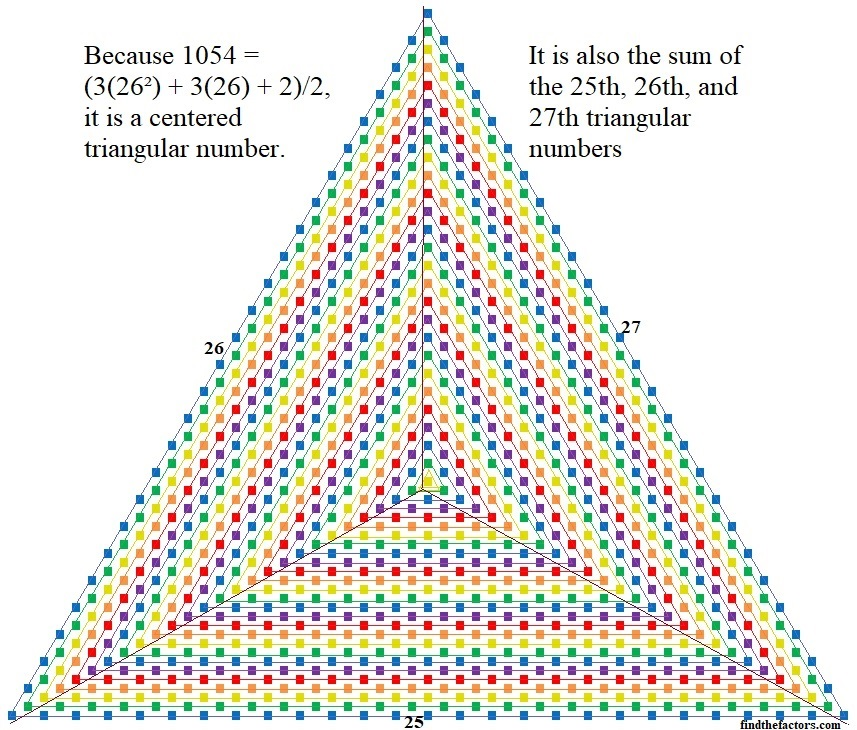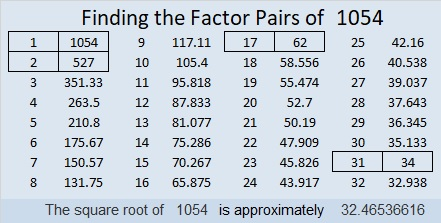# How Can You Count These 1054 Tiny Squares?

There are 1054 tiny squares in the image below, making 1054 a centered triangular number. How can you know that I’m not pulling the wool over your eyes about the number of tiny squares? Here are a few ways that you can quickly count all of them.

If you start with the yellow square in the center and count outward each succeeding triangle you will get 1 yellow square + 3 green squares + 6 blue squares + 9 purple squares + 12 red squares + 15 orange squares, etc. until you reach the final 78 blue squares:
1 + 3 + 6 + 9 + 12 + 15 + . . . + 78
= 1 + 3(1 + 2 + 3 + 4 + 5 + . . . + 26)
= 1 + 3(26*27)/2 = 1 + 3(351) = 1054

Using a little bit of algebra, you can show that
1 + 3(26*27)/2 = (3(26²) + 3(26) + 2)/2 = 1054You can divide the centered triangle above into three triangles as I also did in the graphic. The three triangles represent the 25th, the 26th, and the 27th triangular numbers. Adding them up you get:
25(26)/2 + 26(27)/2 + 27(28)/2 = 325 + 351 + 378 = 1054

• 1054 is a composite number.
• Prime factorization: 1054 = 2 × 17 × 31
• The exponents in the prime factorization are 1, 1, and 1. Adding one to each and multiplying we get (1 + 1)(1 + 1)(1 + 1) = 2 × 2 × 2 = 8. Therefore 1054 has exactly 8 factors.
• Factors of 1054: 1, 2, 17, 31, 34, 62, 527, 1054
• Factor pairs: 1054 = 1 × 1054, 2 × 527, 17 × 62, or 31 × 34
• 1054 has no square factors that allow its square root to be simplified. √1054 ≈ 32.4653661054 is the sum of six consecutive prime numbers:
163 + 167 + 173 + 179 + 181 + 191 = 1054

1054 is the hypotenuse of a Pythagorean triple:
496-930-1054 which is (8-15-17) times 62

1054 looks interesting when it is written in some other bases:
It’s 4A4 in BASE 15 (A is 10 base 10) because 4(15²) + 10(15) + 4(1) = 1054
1C1 in BASE 27 (C is 12 base 10) because 27² + 12(27) + 1 = 1054
VV in BASE 33 (V is 31 base 10) because 31(33) + 31(1) = 31(34) = 1054
V0 in BASE 34 because 31(34) = 1054

This site uses Akismet to reduce spam. Learn how your comment data is processed.